# Tail Recursion Problems with Recursion Recursion is generally

• Slides: 28Tail Recursion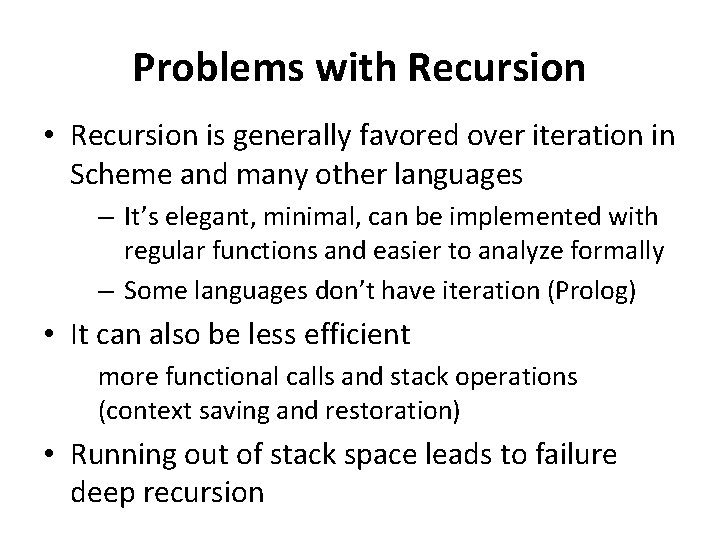Problems with Recursion • Recursion is generally favored over iteration in Scheme and many other languages – It’s elegant, minimal, can be implemented with regular functions and easier to analyze formally – Some languages don’t have iteration (Prolog) • It can also be less efficient more functional calls and stack operations (context saving and restoration) • Running out of stack space leads to failure deep recursionTail recursion is iteration • Tail recursion is a pattern of use that can be compiled or interpreted as iteration, avoiding the inefficiencies • A tail recursive function is one where every recursive call is the last thing done by the function before returning and thus produces the function’s value • More generally, we identify some proceedure calls as tail callsTail Call A tail call is a procedure call inside another procedure that returns a value which is then immediately returned by the calling procedure def foo(data): bar 1(data) return bar 2(data) def foo(data): if test(data): return bar 2(data) else: return bar 3(data) A tail call need not come at the textual end of the procedure, but at one of its logical endsTail call optimization • When a function is called, we must remember the place it was called from so we can return to it with the result when the call is complete • This is typically stored on the call stack • There is no need to do this for tail calls • Instead, we leave the stack alone, so the newly called function will return its result directly to the original caller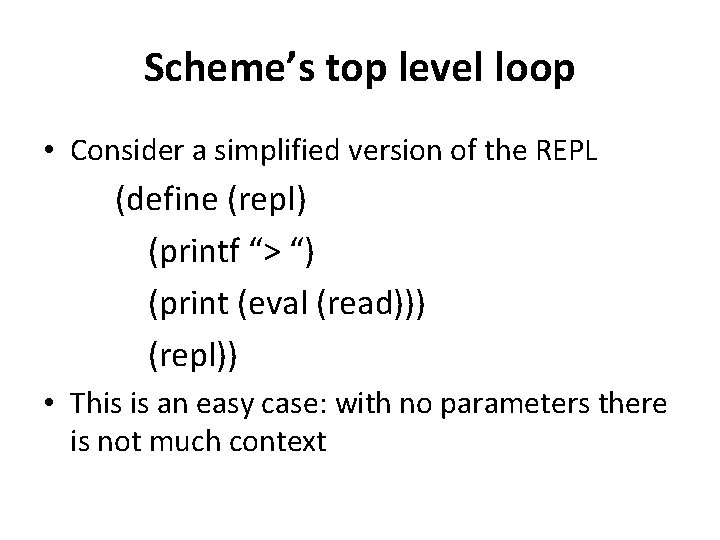Scheme’s top level loop • Consider a simplified version of the REPL (define (repl) (printf “> “) (print (eval (read))) (repl)) • This is an easy case: with no parameters there is not much contextScheme’s top level loop 2 • Consider a fancier REPL (define (repl) (repl 1 0)) (define (repl 1 n) (printf “~s> “ n) (print (eval (read))) (repl 1 (add 1 n))) • This is only slightly harder: just modify the local variable n and start at the top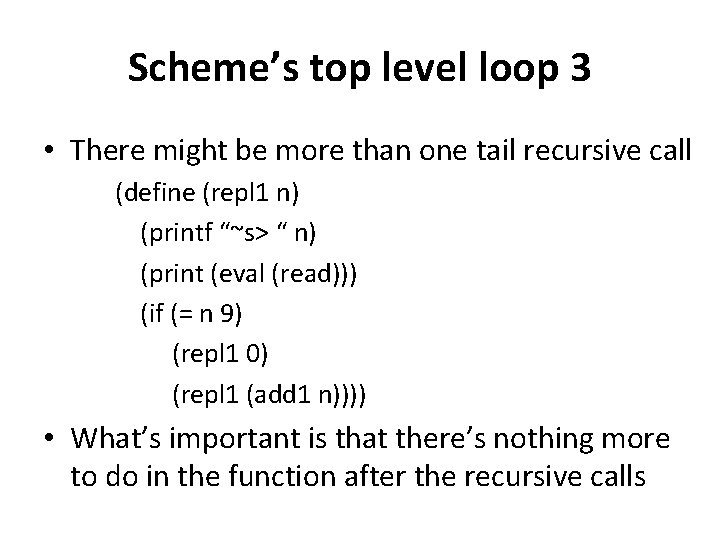Scheme’s top level loop 3 • There might be more than one tail recursive call (define (repl 1 n) (printf “~s> “ n) (print (eval (read))) (if (= n 9) (repl 1 0) (repl 1 (add 1 n)))) • What’s important is that there’s nothing more to do in the function after the recursive calls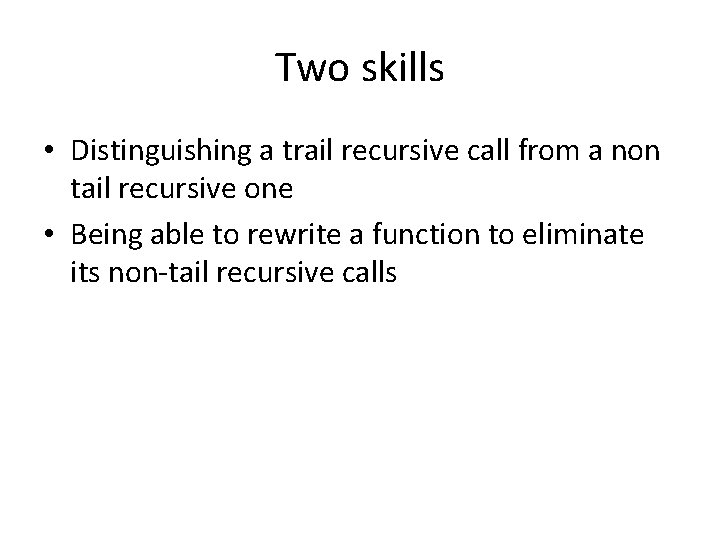Two skills • Distinguishing a trail recursive call from a non tail recursive one • Being able to rewrite a function to eliminate its non-tail recursive calls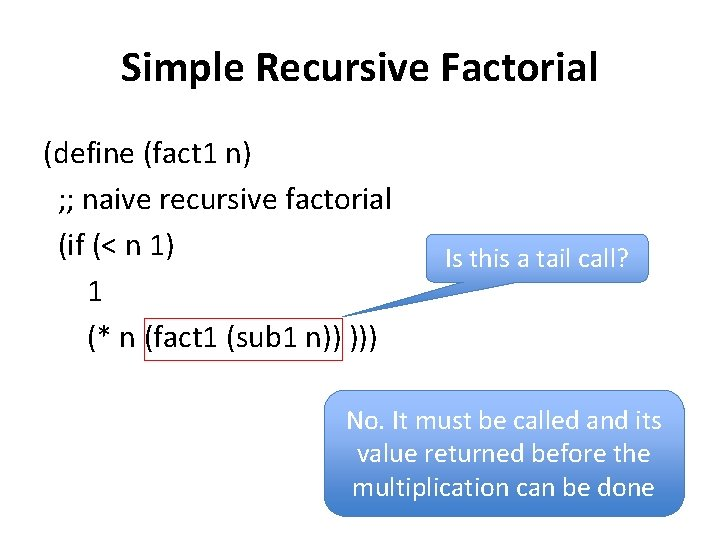Simple Recursive Factorial (define (fact 1 n) ; ; naive recursive factorial (if (< n 1) 1 (* n (fact 1 (sub 1 n)) ))) Is this a tail call? No. It must be called and its value returned before the multiplication can be doneTail recursive factorial (define (fact 2 n) ; rewrite to just call the tail-recursive ; factorial with the appropriate initial values (fact 2. 1 n 1)) Is this a tail call? (define (fact 2. 1 n accumulator) ; tail recursive factorial calls itself ; as last thing to be done Yes. Fact 2. 1’s (if (< n 1) args are evaluaaccumulator ted before it’s (fact 2. 1 (sub 1 n) (* accumulator n)) )) called.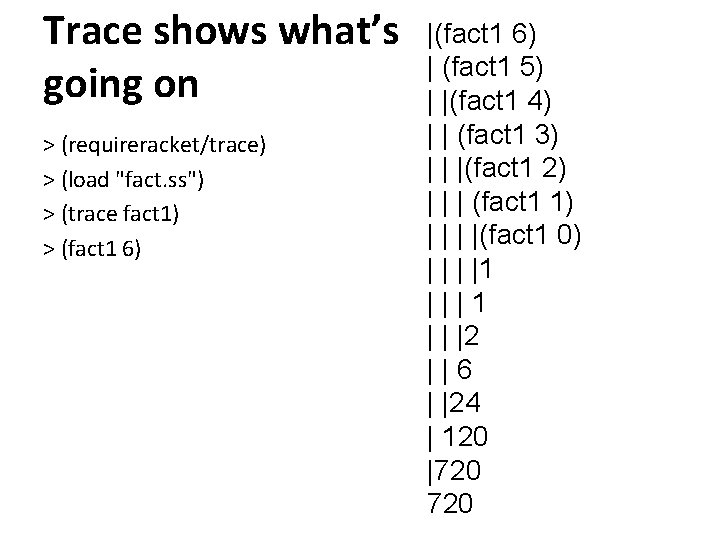Trace shows what’s going on > (requireracket/trace) > (load "fact. ss") > (trace fact 1) > (fact 1 6) | (fact 1 5) | |(fact 1 4) | | (fact 1 3) | | |(fact 1 2) | | | (fact 1 1) | |(fact 1 0) | |1 |||1 | | |2 ||6 | |24 | 120 |720> (trace fact 2. 1) > (fact 2 6) |(fact 2. 1 6 1) |(fact 2. 1 5 6) |(fact 2. 1 4 30) |(fact 2. 1 3 120) |(fact 2. 1 2 360) |(fact 2. 1 1 720) |(fact 2. 1 0 720) |720 fact 2 • Interpreter & compiler note the last expression to be evaled & returned in fact 2. 1 is a recursive call • Instead of pushing state on the sack, it reassigns the local variables and jumps to beginning of the procedure • Thus, the recursion is automatically transformed into iteration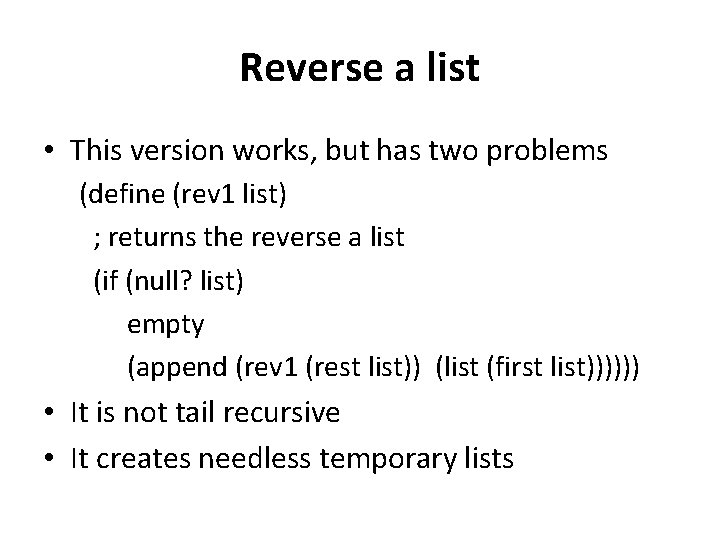Reverse a list • This version works, but has two problems (define (rev 1 list) ; returns the reverse a list (if (null? list) empty (append (rev 1 (rest list)) (list (first list)))))) • It is not tail recursive • It creates needless temporary lists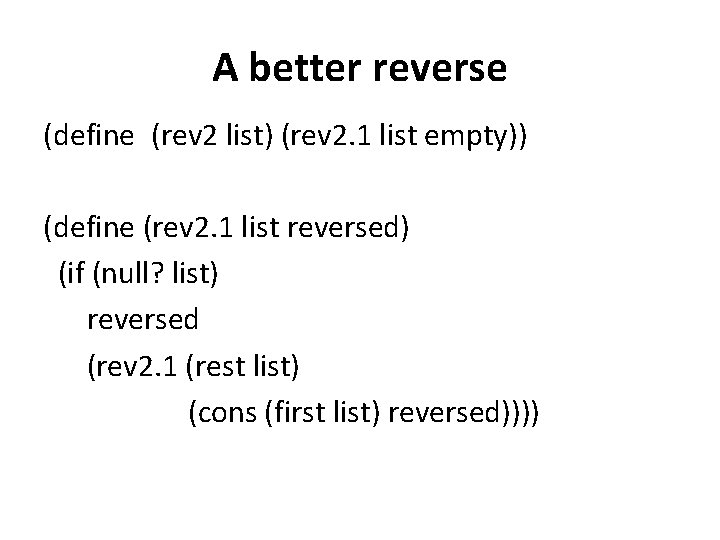A better reverse (define (rev 2 list) (rev 2. 1 list empty)) (define (rev 2. 1 list reversed) (if (null? list) reversed (rev 2. 1 (rest list) (cons (first list) reversed))))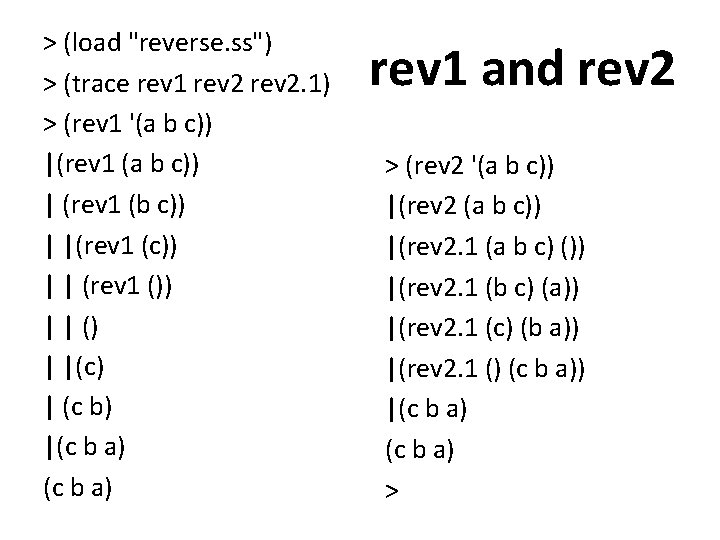> (load "reverse. ss") > (trace rev 1 rev 2. 1) > (rev 1 '(a b c)) |(rev 1 (a b c)) | (rev 1 (b c)) | |(rev 1 (c)) | | (rev 1 ()) | | () | |(c) | (c b) |(c b a) rev 1 and rev 2 > (rev 2 '(a b c)) |(rev 2. 1 (a b c) ()) |(rev 2. 1 (b c) (a)) |(rev 2. 1 (c) (b a)) |(rev 2. 1 () (c b a)) |(c b a) >The other problem • Append copies the top level list structure of it’s first argument. • (append ‘(1 2 3) ‘(4 5 6)) creates a copy of the list (1 2 3) and changes the last cdr pointer to point to the list (4 5 6) • In reverse, each time we add a new element to the end of the list, we are (re-)copying the list.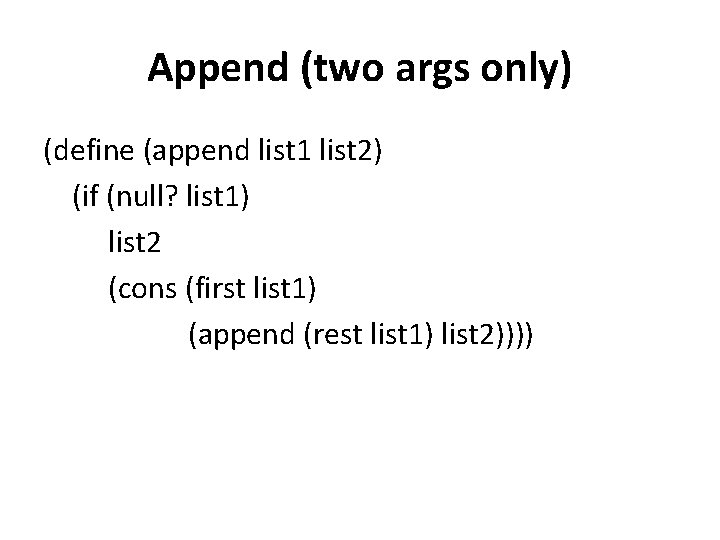Append (two args only) (define (append list 1 list 2) (if (null? list 1) list 2 (cons (first list 1) (append (rest list 1) list 2))))Why does this matter? • The repeated rebuilding of the reversed list is needless work • It uses up memory and adds to the cost of garbage collection (GC) • GC adds a significant overhead to the cost of any system that uses it • Experienced programmers avoid algorithms that needlessly consume memory that must be garbage collectedFibonacci • Another classic recursive function is computing the nth number in the fibonacci series (define (fib n) (if (< n 2) n (+ (fib (- n 1)) (fib (- n 2))))) Are the tail calls? • But its grossly inefficient – Run time for fib(n) ≅ O(2 n) – (fib 100) can not be computed this way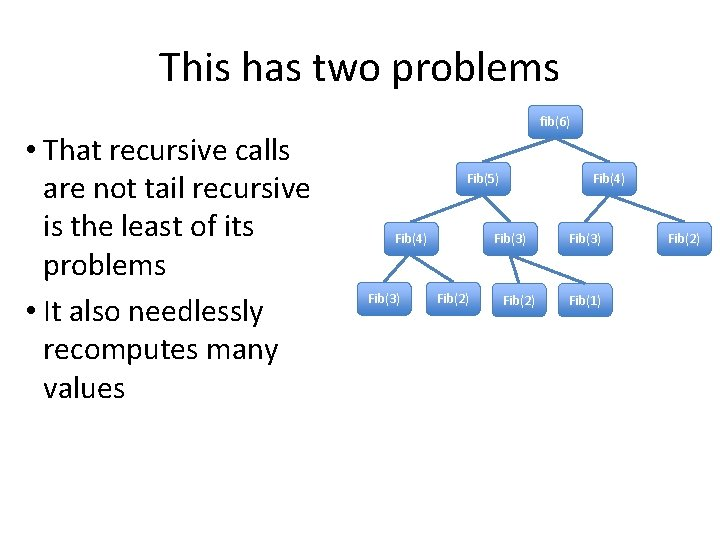This has two problems • That recursive calls are not tail recursive is the least of its problems • It also needlessly recomputes many values fib(6) Fib(5) Fib(4) Fib(3) Fib(2) Fib(3) Fib(1) Fib(2)Tail-recursive version of Fib Here’s a tail-recursive version that runs in 0(n) (define (fib 2 n) (cond ((= n 0) 0) ((= n 1) 1) (#t (fib-tr n 2 0 1)))) (define (fib-tr target n f 2 f 1 ) (if (= n target) (+ f 2 f 1) (fib-tr target (+ n 1) f 1 (+ f 1 f 2)))) We pass four args: n is the current index, target is the index of the number we want, f 2 and f 1 are the two previous fib numbers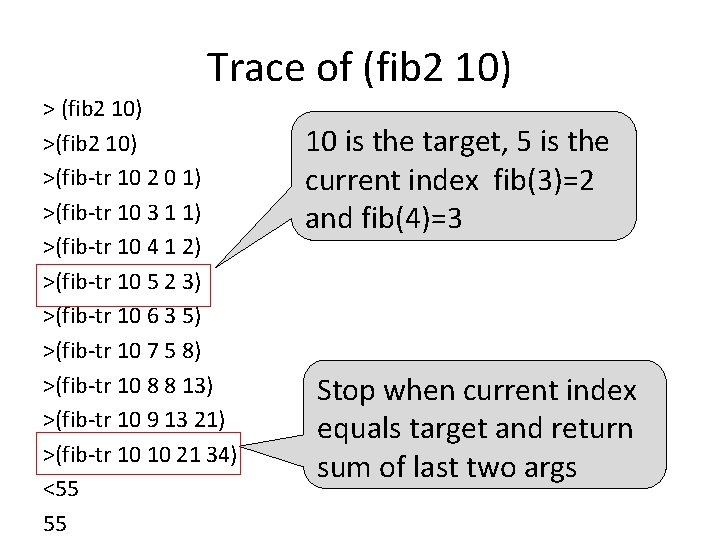Trace of (fib 2 10) >(fib-tr 10 2 0 1) >(fib-tr 10 3 1 1) >(fib-tr 10 4 1 2) >(fib-tr 10 5 2 3) >(fib-tr 10 6 3 5) >(fib-tr 10 7 5 8) >(fib-tr 10 8 8 13) >(fib-tr 10 9 13 21) >(fib-tr 10 10 21 34) <55 55 10 is the target, 5 is the current index fib(3)=2 and fib(4)=3 Stop when current index equals target and return sum of last two argsCompare to an iterative version • The tail recursive version passes the “loop variables” as arguments to the recursive calls • It’s just a way to do iteration using recursive functions without the need for special iteration operators def fib(n): if n < 3: return 1 else: f 2 = f 1 = 1 x=3 while x<n: f 1, f 2 = f 1 + f 2, f 1 return f 1 + f 2No tail call elimination in many PLs • Many languages don’t optimize tail calls, including C, Java and Python • Recursion depth is constrained by the space allocated for the call stack • This is a design decision that might be justified by the worse is better principle • See Guido van Rossum’s comments on TREPython example > def dive(n=1): . . . print n, . . . dive(n+1). . . >>> dive() 1 2 3 4 5 6 7 8 9 10. . . 998 999 Traceback (most recent call last): File "<stdin>", line 1, in <module> File "<stdin>", line 3, in dive. . . 994 more lines. . . File "<stdin>", line 3, in dive Runtime. Error: maximum recursion depth exceeded >>>Conclusion • Recursion is an elegant and powerful control mechanism • We don’t need to use iteration • We can eliminate any inefficiency if we Recognize and optimize tail-recursive calls, turning recursion into iteration • Some languages (e. g. , Python) choose not to do this, and advocate using iteration when appropriate But side-effect free programming remains easier to analyze and parallelize